# 工业设计系教学建议

## 现状

2010级工业设计主要来自第一批科技与创意设计试验班。从第一年接受了部分美术和设计基础课程，但实际上并没有很牢固。这些学生大多数都没有过美术学习经历，只能依样画葫芦，完成作业。而美术基础却是需要时间来反复磨砺的。而由于美术基础课会占用大量时间，科学技术类的课程，如数学、物理，则被大大简化。这对后续的教学产生了深刻影响。

## 教学工作建议

### 增加教师数量

1. 课程数量少，缺乏选择，很多应该跟上时代的课程找不到老师来开
2. 由于课程难以安排，一些老师不得不担任自己不熟悉的课程，课程无法充分开展
3. 部分课程只能启用外聘教师，而外聘教师对浙大工业设计可能并不了解

## 教学资源建议

### 增加必要教室

1. 模型坊，配备操作台和切割打磨等工具
2. 摄影室，摄像器材和幕布、反光板、灯箱
3. 画室，绘画素材和模型，可与其他专业共用
4. 专业教室，每个班应当有一个教室，三个年级六个班，应该有六个专业教室，但现在只有三个，50个人挤在同一个教室

1. 改善网络状况

# Arduino与Processing的串口通信

//Processing Code
import processing.serial.*;

Serial myPort;

void setup(){
myPort = new Serial(this,"/dev/ttyACM0", 115200); //Set Serial Port

}

void draw(){
if(myPort.available()>0){
String message = myPort.readString();
println(message);
}
}

//Arduino code
int data=12345;
void setup()
{
Serial.begin(115200);//rate
}
void loop()
{
Serial.print(data); //send data
delay(1000);
}


12345
123
45
12345
12345


Arduino和Processing的数据收发速度是不一样的。如果用Arduino延时较长时间，Processing可能读取一个字符串或字符串的一部分。如果Arduino延时较短，Processing可能读取多个字符串，但不一定完整。在读取字符串的时候，无法确定上一个字符串是否被读取了，当前字符串是否缓存完毕，因为字符串都已经切成了bytes，连成一串。这个问题是串口通信本身造成的，一定会出现。

Processing的SerialEvent事件类型就提供了这种方式，使用bufferUntil(ch)可以在遇到某个指定字符时才完成缓存。

//Processing Code
import processing.serial.*;

Serial myPort;

void setup(){
myPort = new Serial(this,"/dev/ttyACM0", 115200);  //in fact, any rate is ok...
myPort.bufferUntil('\n');  //buffer until meet '\n', then call the event listener
}

void draw(){

}

//listen to the event. when buffer filled, run this method
void serialEvent(Serial p) {
String inString = p.readString();
print(inString);
}

//then the buffer will reveive all the bytes

//Arduino Code
int data=12345;
void setup()
{
Serial.begin(115200);//rate
}
void loop()
{
Serial.println(data); //send data, end up with '\n'
delay(1000);
}


//Processing Code
import processing.serial.*;

String message;
String temp;
Serial myPort;

void setup(){
myPort = new Serial(this,"/dev/ttyACM0", 115200); //Set Serial Port
}

void draw(){
if(myPort.available()>0){
temp = myPort.readString(); //temp for read bytes
for(int i = 0; i < temp.length(); i++){
//if meet the end mark
if(temp.charAt(i) == '\n'){
println(message);
message = "";  //clean string
}
else
message += temp.charAt(i);  //store byte
}
}
}

//Arduino Code
int data=12345;
void setup()
{
Serial.begin(115200);//rate
}
void loop()
{
Serial.println(data); //send data, end up with '\n'
delay(1000);
}


# 遥控变色灯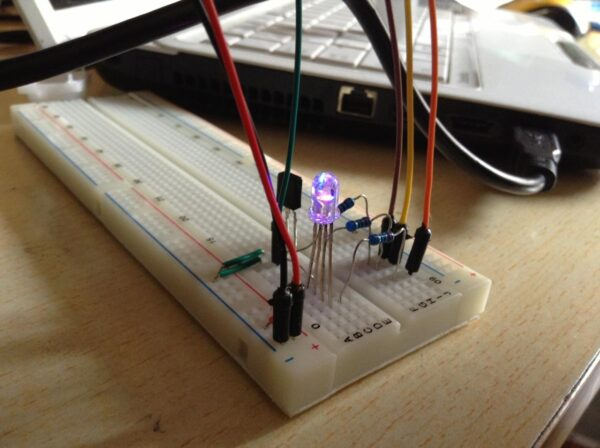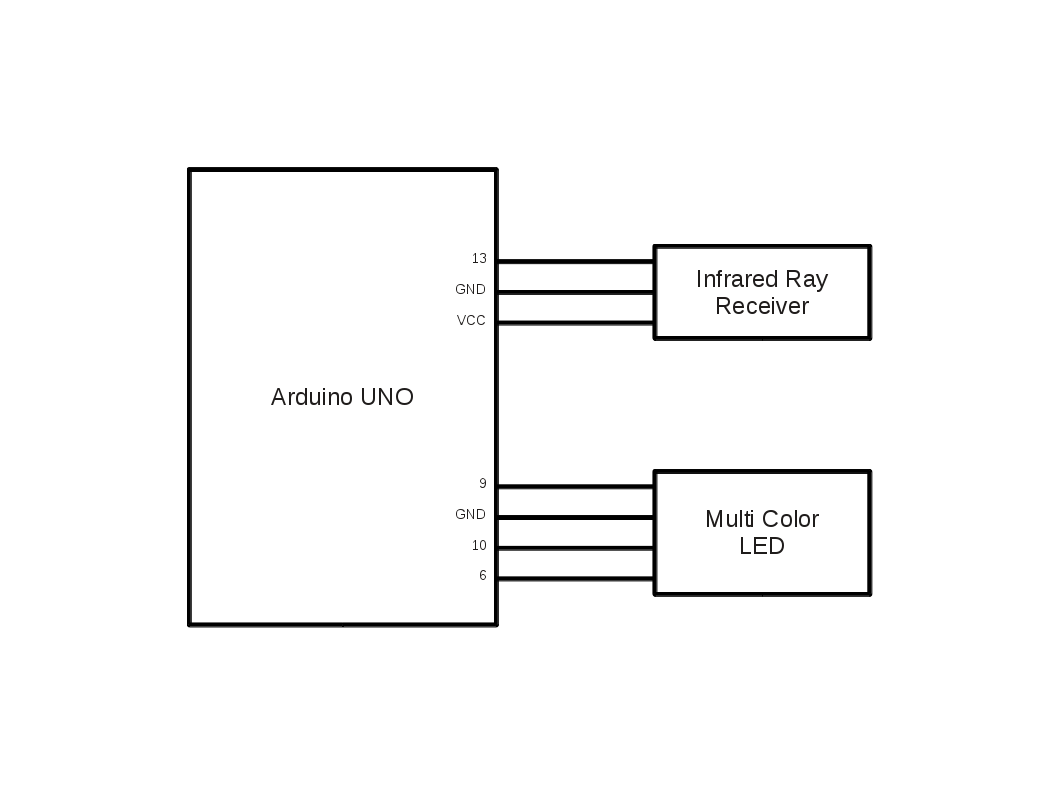Arduino有现成的红外接收库。一开始就非常顺利地接收了红外遥控的信号，并记录下了每个键所对应的值，这是通过串口发送电脑回来的。很特别的是，当一直按下一个键时，后续会重复返回FFFFFFFF。然后根据按键控制红灯的亮度，并设计成若一直按下，则会重复动作，这样就不必按很多次按钮了。

AVR单片机的6路PWM是有差异的。Arduino将其同质化处理，虽然简化了认知过程，但导致很多问题无法解释。机器人协会曾经也讨论过是否要引进Arduino，但后来还是否定了这个计划。因为Arduino的思维方式虽然在早期能够快速入门，但却导致在进阶的过程中处处遭遇困难。以后再去认识硬件细节的设计，摒弃原有的理解，是非常困难的。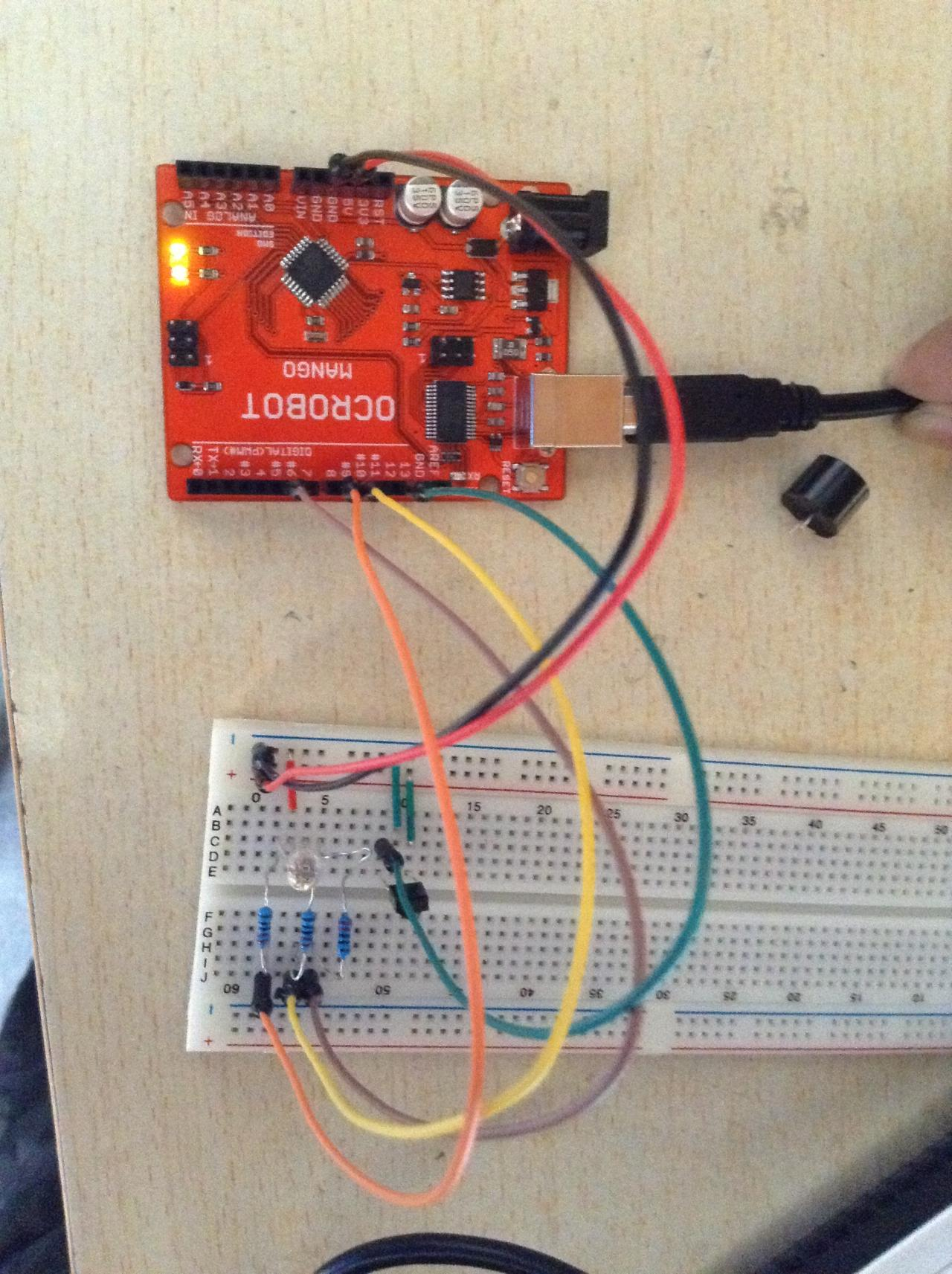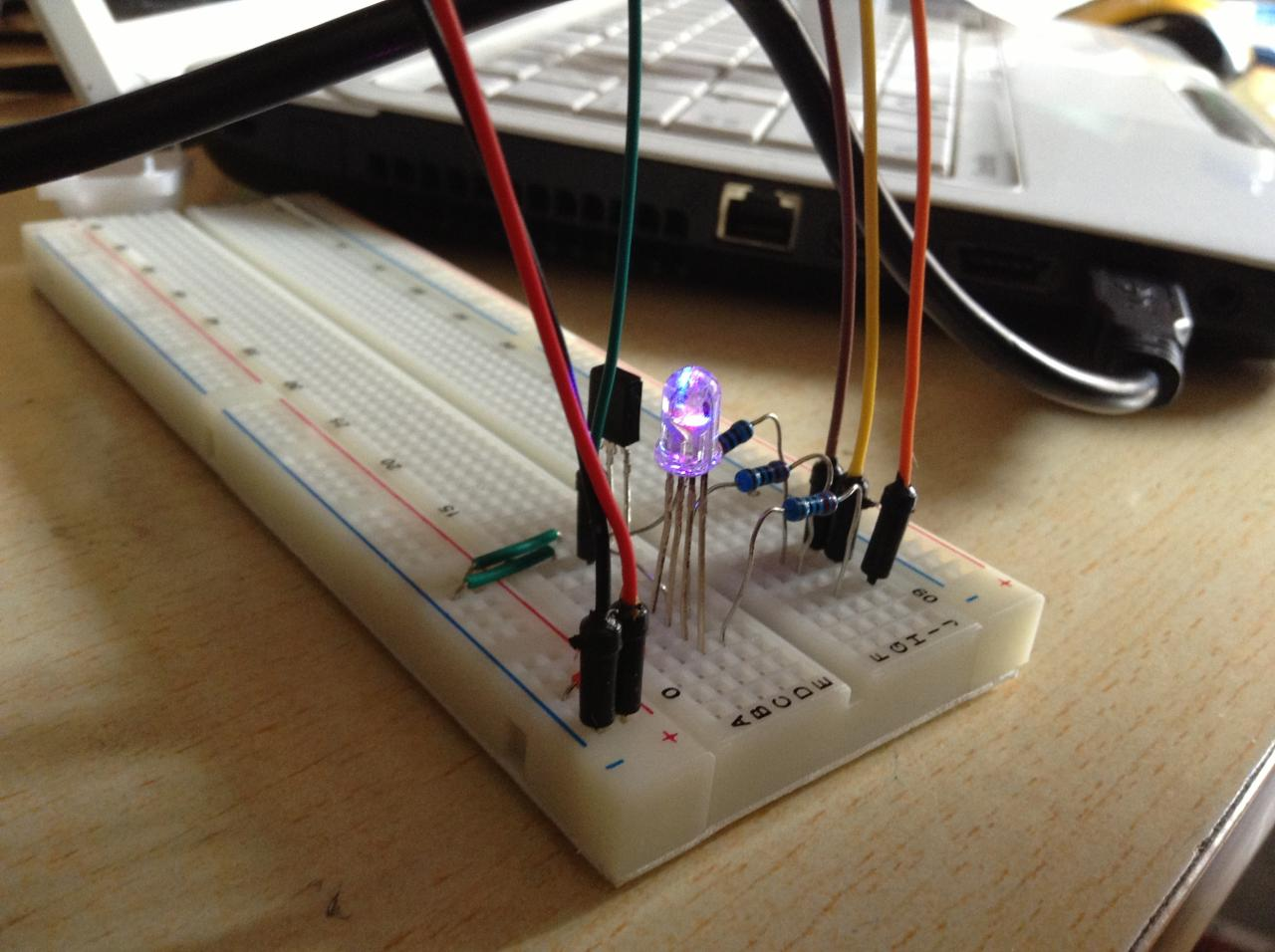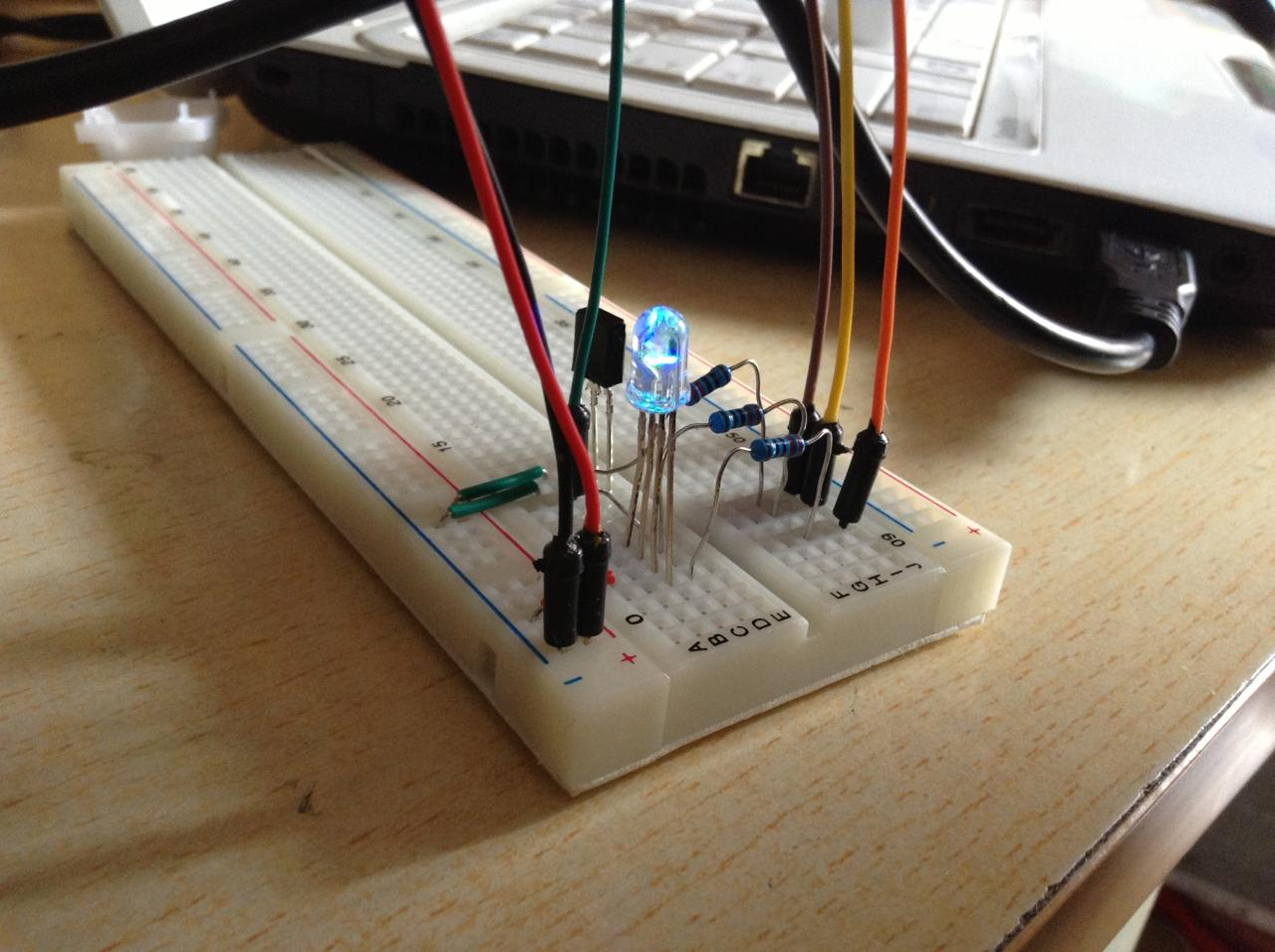#include "IRremote.h"

int RECV_PIN = 13;

int red = 0;
int blue = 0;
int green = 0;

int last = 0;
int now = 0;

IRrecv irrecv(RECV_PIN);

decode_results results;

void setup() {
Serial.begin(9600);
irrecv.enableIRIn();
pinMode(9, OUTPUT);
pinMode(10, OUTPUT);
pinMode(6, OUTPUT);
}

void loop() {

if (irrecv.decode(&results)) {
now = results.value;
Serial.println(now);

if(now==-1)
now = last;

switch(now){
case -23971:
if(red==0)
red=255;
else
red--;
break;
case 25245:
if(red==255)
red=0;
else
red++;
break;
case 8925:
if(green==0)
green=255;
else
green--;
break;
case 765:
if(green==255)
green=0;
else
green++;
break;
case -8161:
if(blue==0)
blue=255;
else
blue--;
break;
case -22441:
if(blue==255)
blue=0;
else
blue++;
break;
case -7651:
red=0;
green=0;
blue=0;
break;
default:break;
}
irrecv.resume();
last = now;
}

color(red, green, blue);
}

void color(int r, int g, int b){
analogWrite(9, r);
analogWrite(10, g);
analogWrite(6, b);
}


# MediaWiki MathJax数学公式

## 原理

MediaWiki的插件一般由php脚本和javascript脚本组成，直接上传到服务器，并在配置文件内添加插件路径即可使用。

Math插件的安装比较繁琐，因为它还依赖于其他本地应用程序，如mineTex。Math是一个统一化的接口，用户使用同样的数学公式代码，却可以通过不同方式生成公式。这些方式包括：PNG图片，LaTex，Tex，HTML，MathML等。而Math并没有实现这些功能的模块，需要调用其它应用程序。

MathJax是一个开源的JavaScript数学公式显示引擎，适用于几乎所有现代浏览器。它被广泛应用于Wiki，WordPress博客等站点。使用它非常简单，只要在网页上的head标签内加入

<script type="text/javascript"
src="http://cdn.mathjax.org/mathjax/latest/MathJax.js?config=TeX-AMS-MML_HTMLorMML"></script>

## 步骤

require_once("$IP/extensions/Math/Math.php"); 第四步，配置Math插件。打开/extensions/Math/Math.php，更改如下几条配置信息： 1.关闭Tex $wgUseTeX = false;

2.开启MathJax

$wgUseMathJax = true; 3.设MathJax为默认 $wgDefaultUserOptions['math'] = MW_MATH_MATHJAX;

Math默认调用MathJax官方网站的引擎。若你在不连接国际互联网的情况下使用，或者希望使用自己的服务器提高效率，可以安装自己的MathJax引擎。

\$wgMathJaxUrl = 'http://yoursite.org/mathjax/MathJax.js?config=TeX-AMS_HTML';

URL修改为你的MathJax引擎所在的位置。